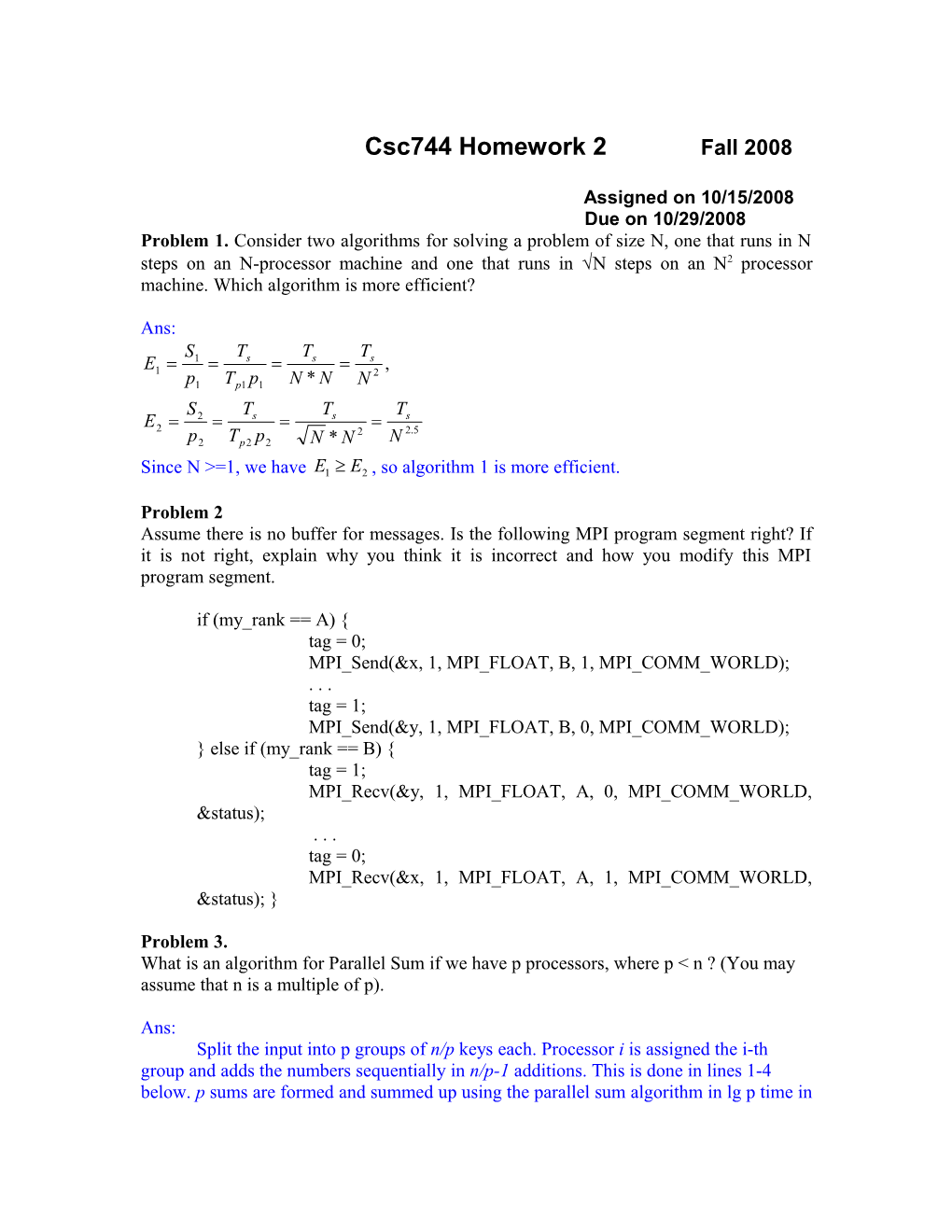# MPI Send(&X, 1, MPI FLOAT, B, 1, MPI COMM WORLD);## Due on 10/29/2008

Problem 1.Consider two algorithms for solving a problem of size N, one that runs in N steps on an N-processor machine and one that runs in N steps on an N2 processor machine. Which algorithm is more efficient?

Ans:

Since N >=1, we have , so algorithm 1 is more efficient.

Problem 2

Assume there is no buffer for messages. Is the following MPI program segment right? If it is not right, explain why you think it is incorrect and how you modify this MPI program segment.

if (my_rank == A) {

tag = 0;

MPI_Send(&x, 1, MPI_FLOAT, B, 1, MPI_COMM_WORLD);

. . .

tag = 1;

MPI_Send(&y, 1, MPI_FLOAT, B, 0, MPI_COMM_WORLD);

} else if (my_rank == B) {

tag = 1;

MPI_Recv(&y, 1, MPI_FLOAT, A, 0, MPI_COMM_WORLD, &status);

. . .

tag = 0;

MPI_Recv(&x, 1, MPI_FLOAT, A, 1, MPI_COMM_WORLD, &status); }

Problem 3.

What is an algorithm for Parallel Sum if we have p processors, where p < n ? (You may assumethat n is a multiple of p).

Ans:

Split the input into p groups of n/p keys each. Processor i is assigned the i-th group and adds the numbers sequentially in n/p-1 additions. This is done in lines 1-4 below. p sums are formed and summed up using the parallel sum algorithm in lg p time in step 5. Total is n/p + lg p - 1. If n is not a multiple of p and lg p is not an integer, two more steps are required.

Sum(n,p,A[0..n-1])

1 mypid = pid(); //pid = 0...p-1

2 sum[pid]=0;

3 for(i=0;i<n/p-1;i++)

4 sum[pid] = sum[pid] + A[pid*n/p+i];

5 ParallelSum(+,sum,Sum); //Parallel sum on p inputs

6 Return(Sum)

Problem 4.You are given n binary values X1,…, Xn. Find the logical OR of these n values in constant time on a CRCWPRAM with n processors.

Ans:

The result stored in cell 0 is 0 (TRUE) unless a processor writes a 1 in cell 0; then one of the Xi is 1 (TRUE) and the result X should be TRUE, as it is.

The algorithm is the following:

begin Logical OR (X1 . . .Xn)

1. Proc 1 writes 0 in cell 0.

2. if Xi = 1 processor i writes 1 into cell 0.

end Logical OR

Problem 5.

How fast can you evaluate in parallel xn for some input x and integer n? Express running time, number of processors used and parallel work in terms of n. Your algorithm must minimize all three parameters (for you to receive full credit).

Ans:

The sequential O(lg n) algorithm is hard to beat asymptotically.

A. Uniprocessor case. The algorithm works as follows. First compute all powers , for all,and if xn is none of them, find the binary representation of n. Then form a product of all 's for whichthe j-th bit in the binary representation of n is an 1. This will get you xn. For example, .

Power( x, n)

1. k = floor(lgn); X=x;

2. for (i=1;i<=k;i++)

3. X[i] = X[i-1]*X[i-1] // Form all powers X[i]=x**(2^i)

4. n= B[k]B[k-1]...B //binary representation of n

5. if (B == 1) P=X;

6. else P=1;

7. for(i=1;i<=k;i++);

8. if (B[i]==1) P= P* X[i]; //multiply all power X[i] for which B[i] is an one

9. return(P); //Done

If you analyze carefully the algorithm above running-time is . P = 1, W = W2 = θ(lg n),T = .

B. Two-processor case.

What if we have two processors? One can (almost) halve the time as follows: One of the two processorscomputes the (or the X[i] terms in our pseudocode), one per step, and a second processor decides,based on bit manipulations of n, whether to include in the product that will compute xn. So oneprocessor does lines 2-3 and the other lines 7-8 of the sequential Power; everything works fine becausethe computation of the two processors is interlaced (i.e. one step of one, is followed by one step of the

other). We show this program Power_2 in the following pseudocode.

Running time of Power_2 is T = + 1. But we use two processors. P = 2, W = W2 = θ (lg n),T = + 1.

Power_2 (x,n)

Step Proc 0| Proc 1

0: X=x | P ; n;

1 : X = x**(2**1) | x=X;

| if (LSB(n)==1) P=x //Determine B

| else P=1

| n=SHR(n);

| if (n==0) return(P);

2 : X=X*X | if (LSB(n)==1) P= P*X;//Determine B

// X= x**(2**2) | n=SHR(n);

| if (n==0) return(P);

|

.....

|

i : X[i]=X[i-1]*X[i-1] | if (LSB(n)==1) P= P*X[i-1];//DetermineB[i-1]

// X[i]= x**(2**i) | n=SHR(n);

| if (n==0) return(P);

|

|

k=lgn : X[k]=X[k-1]*X[k-1] | if (LSB(n)==1) P= P*X[k-1];//Det. B[k-1]

// X[k]= x**(2**k) | n=SHR(n)

| if (n==0) return(P)

|

k+1 : No-operation | if (LSB(n)==1) P= P*X[k]; //Det B[k]

return(P);

Can we do exponentiation faster or with less work?

Any other parallel algorithm will do more work, or spend more time.

(Not required, but) One can prove by a simple inductive argument, if only multiplications are used inthe computation of xn, that in the i-th step all powers up to can only be computed. Thus xn requiresat least lg n steps.